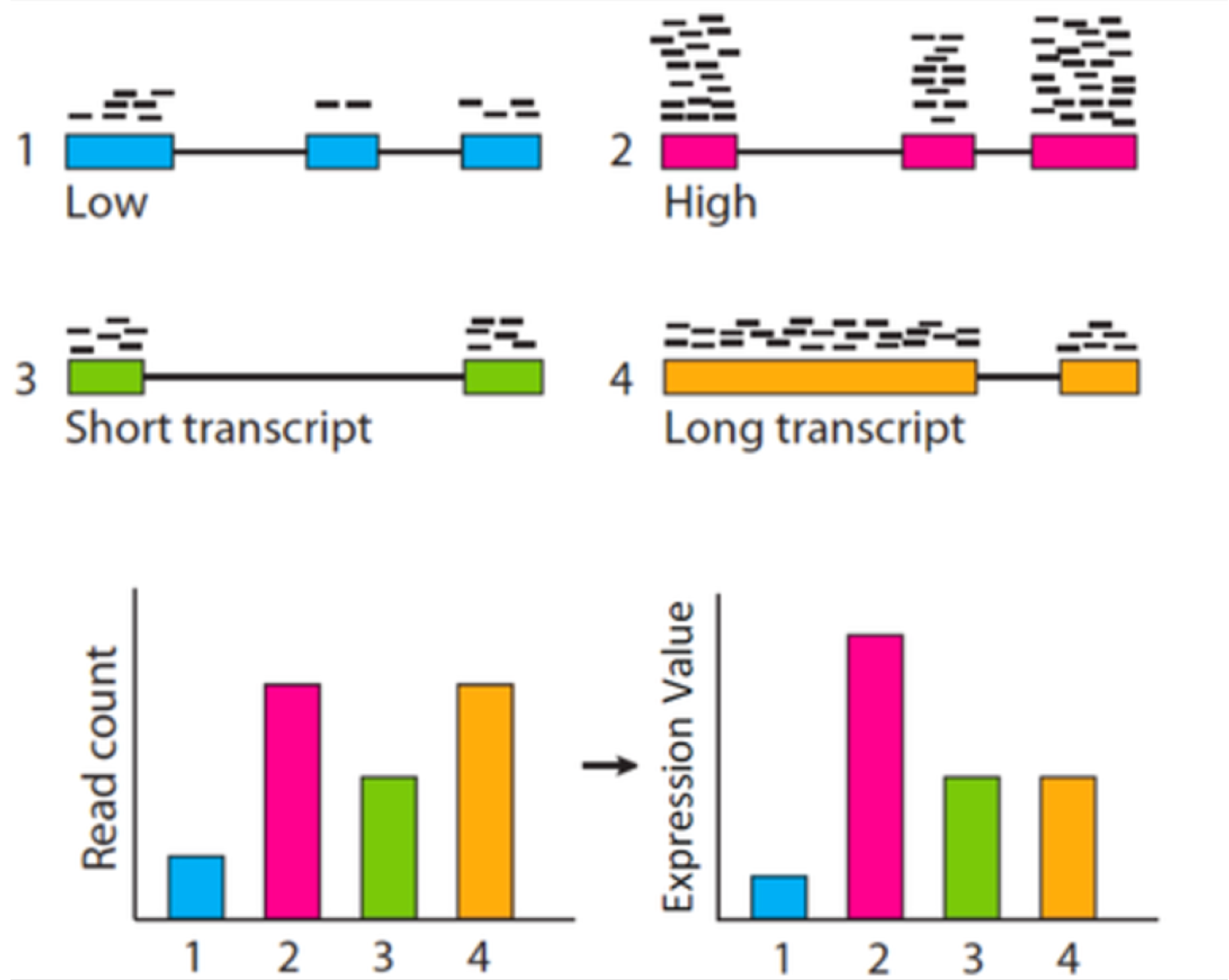#DAY 14
0

## RNA-Sick@Day14 > 檢定只是裝飾而已，上面的大人物是不會懂的｜篩選表現量有顯著差異的基因 feat. edgeR

### 超級比一比之 CPM, FPKM, TPM

RNA-Seq 的主要目的之一就是找到試驗設計處理組間的差異表現基因 (Differential Expression Genes, DEG)，這些基因之所以差異表現的生物意義再交由下游分析或是實際的分子生物實驗探討。先前已經介紹了 alignment-based 和 alignment-free 的轉錄產物定量工具，如：Bowtie2 和 kallisto，一個轉錄產物的定量結果可以用 counts per million (CPM) 來表示，這就是該轉錄產物豐富度的觀測值，下一步就是透過統計檢定了解該轉錄產物的豐富度在處理組間是否有顯著差異。統計檢定挑出差異表現基因這一步通常都可以透過 R 套件完成，例如 edgeR, DESeq2 等等。(看點療癒的東西放空一下，截圖來自龍貓)

### edgeR 源代碼的部分

``````# 參考資料 https://gist.github.com/jdblischak/11384914
setwd("~/Desktop")
source("http://bioconductor.org/biocLite.R")
# biocLite("edgeR", dependencies = TRUE)
library(edgeR)
dim(data_raw)
tail(data_raw)
rownames(data_raw) <- data_raw\$id
data_clean <- data_raw[1:(nrow(data_raw)), 2:(ncol(data_raw))]
#{r expr-cutoff}
data_clean
cpm_log <- cpm(data_clean, log = TRUE)
median_log2_cpm <- apply(cpm_log, 1, median)
hist(median_log2_cpm)
expr_cutoff <- -1
abline(v = expr_cutoff, col = "red", lwd = 3)
sum(median_log2_cpm > expr_cutoff) ##看看還剩下多少有過門檻
data_clean <- data_clean[median_log2_cpm > expr_cutoff, ]

# After removing all genes with a median log~2~ cpm below `r expr_cutoff`, we have `r sum(median_log2_cpm > expr_cutoff)` genes remaining.
# A good rule of thumb when analyzing RNA-seq data from a single cell type is to expect 9-12 thousand expressed genes.
# In this case, we have many more because the samples include RNA collected from many different cell types, and thus it is not surprising that many more genes are robustly expressed.
# **Question:**
#   What are some ways that we could improve this simple cutoff?
# What information are we ignoring?
# After filtering lowly expressed genes, we recalculate the log~2~ cpm.

cpm_log <- cpm(data_clean, log = TRUE)
# ```
# We can also explore the relationships between the samples by visualizing a heatmap of the correlation matrix.
# The heatmap result corresponds to what we know about the data set.
# First, the samples in group A and B come from very different cell populations, so the two groups are very different.
# Second, since the samples in each group are technical replicates, the within group variance is very low.
# ```{r heatmap}
heatmap(cor(cpm_log))
# ```
# Another method to view the relationships between samples is principal components analysis (PCA).
# ```{r pca}
pca <- prcomp(t(cpm_log), scale. = TRUE)
plot(pca\$x[, 1], pca\$x[, 2], pch = ".", xlab = "PC1", ylab = "PC2")
text(pca\$x[, 1], pca\$x[, 2], labels = colnames(cpm_log))
summary(pca)

## Two group comparison
# Now we start preparing the data for the the test of differential expression.
# We create a vector called, `group`, that labels each of the columns as belonging to group A or B.
# We then use this vector and the gene counts to create a DGEList, which is the object that edgeR uses for storing the data from a differential expression experiment.
# ```{r make-groups-edgeR}
group <- substr(colnames(data_clean), 1, 1)
group # 分組的代號
y <- DGEList(counts = data_clean, group = group)
y
# ```
# edgeR normalizes the genes counts using the method TMM (trimmed means of m values) developed by `r citet(c(Robinson2010="10.1186/gb-2010-11-3-r25"))`.
# Recall from lecture that the read counts for moderately to lowly expressed genes can be strongly influenced by small fluctuations in the expression level of highly expressed genes.
# In other words, small differences in expression of highly expressed genes between samples can give the appearance that many lower expressed genes are differentially expressed between conditions.
# TMM adjusts for this by removing the extremely lowly and highly expressed genes and also those genes that are very different across samples.
# It then compares the total counts for this subset of genes between the two samples to get the scaling factor (this is a simplification).
# Similar to normalization methods for microarray data, this method assumes the majority of genes are not differentially expressed between any two samples.
# ```{r normalize-edgeR}
y <- calcNormFactors(y)
y\$samples
# ```
# The next step is to model the variance of the read counts per gene.
# A natural method for modeling gene counts is the Poisson distribution.
# However, the Poisson assumes the mean and variance are identical, but it has been found empirically that the variance in RNA-seq measurements of gene expression are larger than the mean (termed "overdispersion").
# So instead the negative binomial distribution is used, which has a dispersion parameter for modeling the increase in variance from a Poisson process.
# edgeR treats the Poisson variance as simple sampling variance, and refers to the dispersion estimate as the "biological coefficient of variation."
# Though it should be mentioned that any technical biases are also included in this estimate.
# edgeR shares information across genes to determine a common dispersion.
# It extends this to a trended dispersion to model the mean-variance relationship (lowly expressed genes are typically more noisy).
# Lastly it calculates a dispersion estimate per gene and shrinks it towards the trended dispersion.
# The gene-specific (referred to in edgeR as tagwise) dispersion estimates are used in the test for differential expression.
# ```{r model-edgeR}
y <- estimateDisp(y)
sqrt(y\$common.dispersion) # biological coefficient of variation
plotBCV(y)
# ```
# The biological coefficient of variation is lower than normally seen in human studies (~0.4) because the samples are technical replicates.
# edgeR tests for differential expression between two classes using a method similar in idea to the Fisher's Exact Test.
# {r test-edgeR}

et <- exactTest(y)
results_edgeR <- topTags(et, n = nrow(data_clean), sort.by = "none")
results_edgeR
# How many genes are differentially expressed at an FDR of 10%?
# ```{r count-de-edgeR}
sum(results_edgeR\$table\$FDR < .1)
plotSmear(et, de.tags = rownames(results_edgeR)[results_edgeR\$table\$FDR < .1])
abline(h = c(-2, 2), col = "blue")
# ```
# As expected from the description of the samples and the heatmap, there are many differentially expressed genes.
# The [MA plot][ma] above plots the log~2~ fold change on the y-axis versus the average log~2~ counts-per-million on the x-axis.
# The red dots are genes with an FDR less than 10%.
# The blue lines represent a four-fold change in expression.
# [ma]: https://en.wikipedia.org/wiki/MA_plot
``````

### 題外話之沒有重複怎麼辦(在命運的安排下有時候還是會要做這種分析，截圖來自 JoJo 的奇妙冒險)

### 參考資料與延伸閱讀

TPM data Differential expression analysis

Differential expression analysis starting from TPM data

RNAseq基因表現量常用指標：RPKM, FPKM,TPM

### 1 則留言

0

RNA-Sick iT邦新手 5 級 ‧ 2020-02-20 15:44:06 檢舉